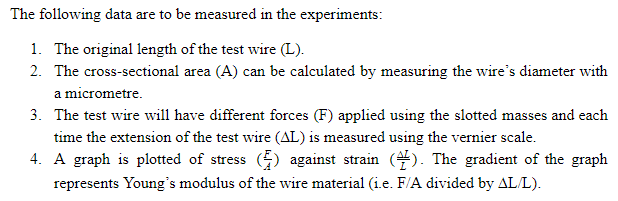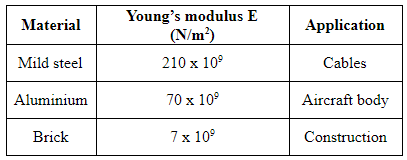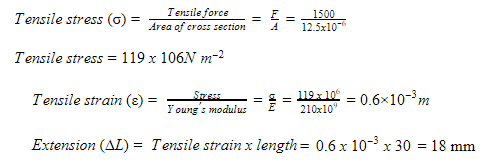# Young’s Modulus

Introduction

Young’s modulus is a numerical constant, named after the 18th-century English physician and physicist Thomas Young. Young’s modulus is a measure of the ability of a material to withstand changes in length under lengthwise tension or compression. Young’s modulus is also termed the modulus of elasticity.

A. Young’s modulus

Most materials under small strain obey Hooke’s law. Under this circumstance, the ratio between stress and strain is constant. This quantity is called Young’s modulus (E). Young’s modulus measures the resistance of a material to elastic deformation.B. What Young’s Modulus Means

Young’s modulus describes the stiffness of a material. In simpler terms, it states how easy it is to bend or stretch the material. Young’s modulus of a material is its fundamental property that remains unchanged. But, it is dependent on material temperature. The stress-strain graph for two materials is illustrated in Figure 1. Material A is stiffer than material B, as a higher stress is needed to produce the same amount of strain. So, Material A will have higher Young’s modulus than Material B.Figure 1: Young’s Modulus Comparison

C. How to Calculate Young’s Modulus

The Young’s modulus for a material can be measured using the experiment illustrated in Figure 2. The reference wire and test wire made of the selected material are hung from the ceiling. The reference wire supports a vernier scale, which will measure the extension of the test wire. The slotted masses can be used to vary the force acting on the test wire.Figure 2: Experiment Set-upD. Applications of Young’s Modulus

Young’s modulus is very important for doctors and scientists. This parameter can help them determine when a structural implant will deform. Hence, they can design a piece mechanically for use in the body. The following table shows Young’s modulus for different values and applications of the materials.An Example of Application

A steel wire of diameter 4.0 mm (Area = 12.5 x 10^(-6) m^2) and length 30 m is suspended from an overhead crane and a load of 1500 N is suspended from its free end. The Young’s modulus of the material of the wire is 210 x 10^9 N. It is assumed that the proportionality limit of the wire is not exceeded. The stress, strain and the extension of the wire used in the overhead crane are calculated.Summary

Young’s modulus is an important material property because of its relationship with stress and strain. Young’s modulus for almost all materials is established. In several engineering design calculations, Young’s modulus is used to find either thickness of the material to withstand a given load or stress level on the material for a given load.